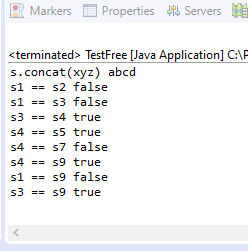### Important Interview Questions of String == and equals | String Code Interview Question | String Interview question Test cases

Here some basic string interview question which has asked by interview. Many interviews you will face this string test scenario which can be asked by the interviewer. If you go all these cases you will able to you know no string double equals questionsString Code Interview Question

```public class StringTestCases {
public static void main(String[] args) {

String s = "abcd";
s.concat("xyz");
System.out.print("s.concat(xyz) ");
System.out.println(s); // abcd

String s1 = new String("abcd");
String s2 = new String("abcd");
System.out.print("s1 == s2 ");
System.out.println(s1 == s2);

String s3 = "abcd";
System.out.print("s1 == s3 ");
// false one object in heap memory and Second in Scp
System.out.println(s1 == s3);

String s4 = "abcd";
System.out.print("s3 == s4 ");
System.out.println(s3 == s4); // True

String s5 = "ab" + "cd";
System.out.print("s4 == s5 ");
System.out.println(s4 == s5); // True

String s6 = "ab";
String s7 = s6 + "cd";
System.out.print("s4 == s7 ");
System.out.println(s4 == s7); // false

final String s8 = "ab";
String s9 = s8 + "cd";
System.out.print("s4 == s9 ");
System.out.println(s4 == s9); // true

System.out.print("s1 == s9 ");
System.out.println(s1 == s9); // false

System.out.print("s3 == s9 ");
System.out.println(s3 == s9); // true
}

}
```

This is the Output of above code.Questions of String == equals